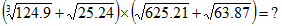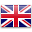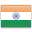Home » Quizzes & Surveys » Quantitative Aptitude – Approximation Quiz-1

# Quantitative Aptitude – Approximation Quiz-1

Welcome to your Quantitative Aptitude Approximation Quiz -1

Calculate the Approximate Value:
25.21 − 212.02 ÷ 38.87= 8 × ?Deselect Answer

Calculate the Approximate Value :EnglishHindi